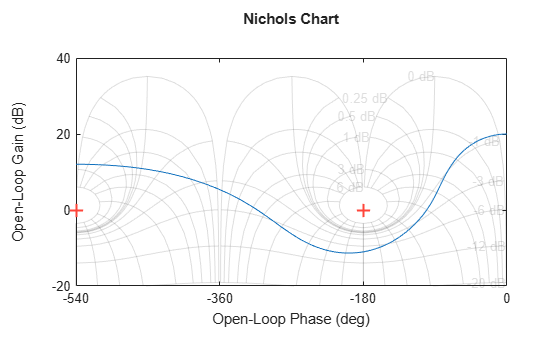# ngrid

Superimpose Nichols chart on Nichols plot

## Syntax

``ngrid``
``ngrid('new')``
``ngrid(AX,___)``

## Description

example

````ngrid` superimposes Nichols chart grid lines over the Nichols frequency response of a SISO LTI system. The range of the Nichols grid lines is set to encompass the entire Nichols frequency response.The chart relates the complex number H/(1 + H) to H, where H is any complex number. For SISO systems, when H is a point on the open-loop frequency response, then the corresponding value of the closed-loop frequency response assuming unit negative feedback is$\frac{H}{1+H}$If the current axis is empty, `ngrid` generates a new Nichols chart grid in the region –40 dB to 40 dB in magnitude and –360 degrees to 0 degrees in phase. `ngrid` returns a warning if the current axis does not contain a SISO Nichols frequency response.```
````ngrid('new')` clears the current axes first and sets ```hold on```.```
````ngrid(AX,___)` plots the grid on the `Axes` or `UIAxes` object in the current figure with the handle `AX`. Use this syntax when creating apps with `ngrid` in the App Designer.```

## Examples

collapse all

Plot the Nichols response with Nichols grid lines for the following system:

`$H\left(s\right)=\frac{-4{s}^{4}+48{s}^{3}-18{s}^{2}+250s+600}{{s}^{4}+30{s}^{3}+282{s}^{2}+525s+60}.$`

```H = tf([-4 48 -18 250 600],[1 30 282 525 60]); nichols(H) ngrid```The context menu for Nichols charts includes the Tight option under Zoom. You can use this option to clip unbounded branches of the Nichols chart.

## Input Arguments

collapse all

Object handle, specified as an `Axes` or `UIAxes` object. Use `AX` to create apps with `ngrid` in the App Designer.

## Version History

Introduced before R2006a